iOS - 揭露Block的内部实现原理

抛砖引玉

void blockFunc1()
{
int num = 100;
void (^block)() = ^{
NSLog(@"num equal %d", num);
};
num = 200;
block();
}
void blockFunc2()
{
__block int num = 100;
void (^block)() = ^{
NSLog(@"num equal %d", num);
};
num = 200;
block();
}

blockFunc1 : num equal 100
blockFunc2 : num equal 200

// 全局变量
int num = 100;
void blockFunc3()
{
void (^block)() = ^{
NSLog(@"num equal %d", num);
};
num = 200;
block();
}
void blockFunc4()
{
static int num = 100;
void (^block)() = ^{
NSLog(@"num equal %d", num);
};
num = 200;
block();
}

探索内部原理

Objective-C是一个全动态语言，它的一切都是基于runtime实现的！在运行时会将OC转换成C，我们可以利用这个来查看关于block在内部是如何实现的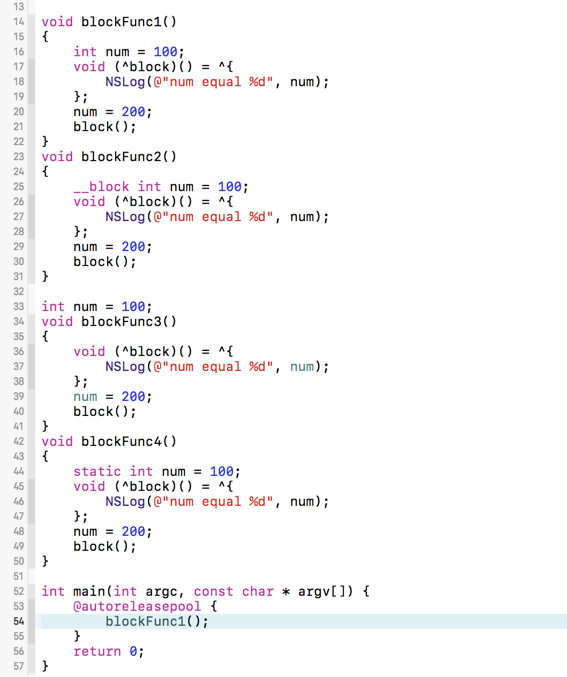clang -rewrite-objc main.m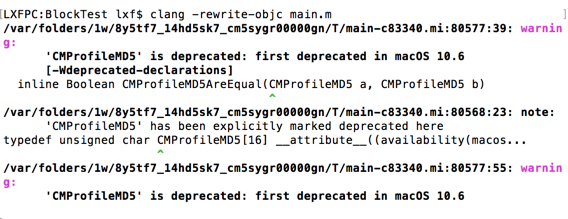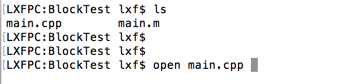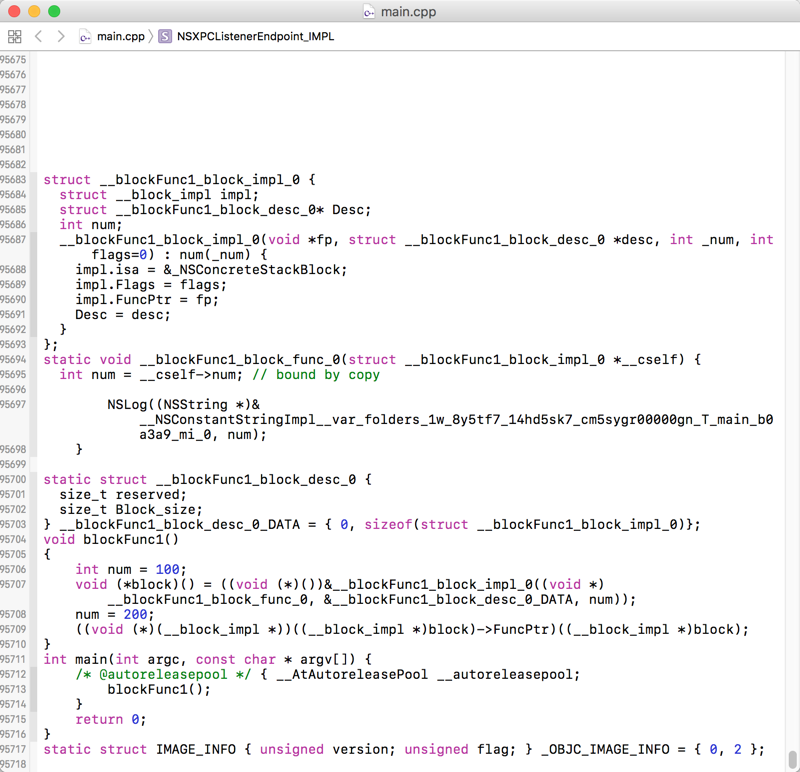void blockFunc1()
{
int num = 100;
void (*block)() = ((void (*)())&__blockFunc1_block_impl_0((void *)__blockFunc1_block_func_0, &__blockFunc1_block_desc_0_DATA, num));
num = 200;
((void (*)(__block_impl *))((__block_impl *)block)->FuncPtr)((__block_impl *)block);
}

void blockFunc1()
{
int num = 100;
// *************************重点句***********************
void (*block)() = &__blockFunc1_block_impl_0(__blockFunc1_block_func_0, &__blockFunc1_block_desc_0_DATA, num));
// *****************************************************
num = 200;
((void (*)(__block_impl *))((__block_impl *)block)->FuncPtr)((__block_impl *)block);
}

block实际上是指向结构体的指针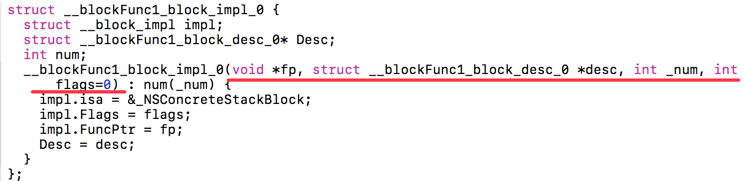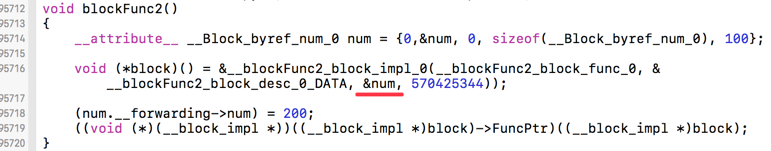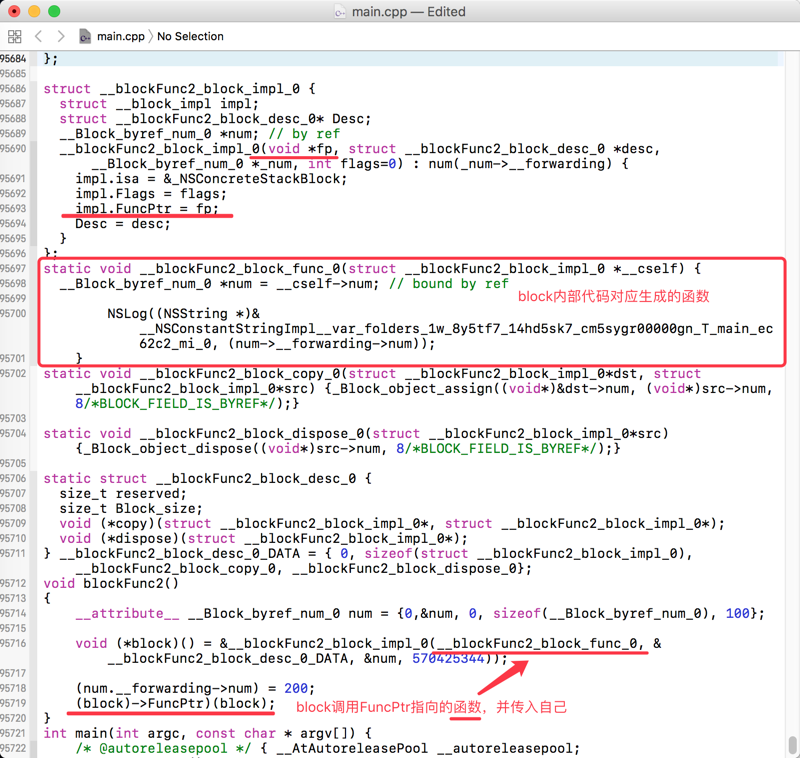* SO *

__block的实现

struct __Block_byref_num_0 {
void *__isa;  // isa指针
__Block_byref_num_0 *__forwarding;  // 实例本身
int __flags;
int __size;
int num;  // 我们的num值
};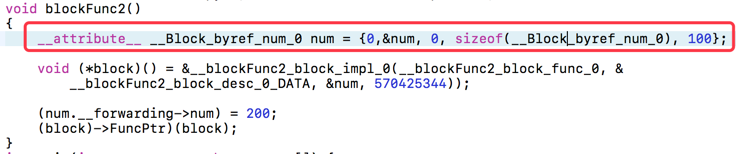__block int num = 100;

num = 200;

(num.__forwarding->num) = 200;